# B.2. Instance Diagrams

A UML instance diagram, also known as a UML object diagram, shows a snapshot of the state of one or more objects. These diagrams are used extensively in Part I of this book, and are crucial to understanding the linked structures introduced in Chapter 6.

More than one instance of a class may be shown in an instance diagram (Figure B-8). Static fields and all methods are omitted, as they are the same for every instance. The name of the class of each instance is underlined, but not bold. A specific value is given for each primitive field.

Figure B-8. UML instance diagram showing two instances of the Starship class.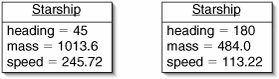References are shown as arrows. In Figure B-9, the instance of class Outfit has four fields: price, top, bottom, and feet. Technically speaking, fields of type String are references to instances of the String class, but we omit this detail for clarity.

Figure B-9. An instance of class Outfit with references to three other objects.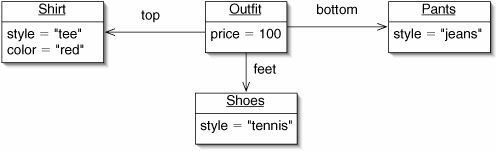A number of minor extensions to standard UML instance diagrams are used in this book.

First, a null reference is sometimes shown as a line ending in a large dot (Figure B-10). If including such lines would complicate the diagram excessively, null references are simply omitted.

Figure B-10. In this instance of class Outfit, the feet field contains a null reference.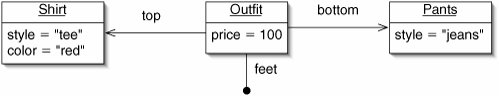Second, arrays are shown as rows of boxes (Figure B-11). Each box may contain a primitive value or a reference to an object or array.

Figure B-11. This instance of Menu contains two arrays, prices and items.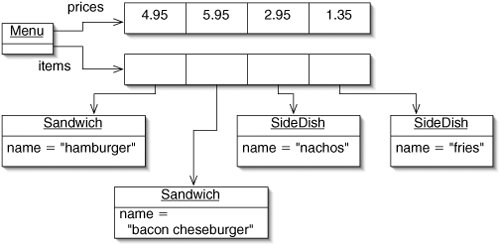Third, variables, arguments, and constants are sometimes included in UML instance diagrams (Figure B-12).

Figure B-12. The variable target has the value 17. The constant ORIGIN is a reference to an instance of Point.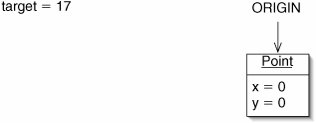We end this appendix by noting that some special notation is used in Chapter 16, which deals with references in great detail.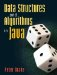Data Structures and Algorithms in Java
ISBN: 0131469142
EAN: 2147483647
Year: 2004
Pages: 216
Authors: Peter Drake